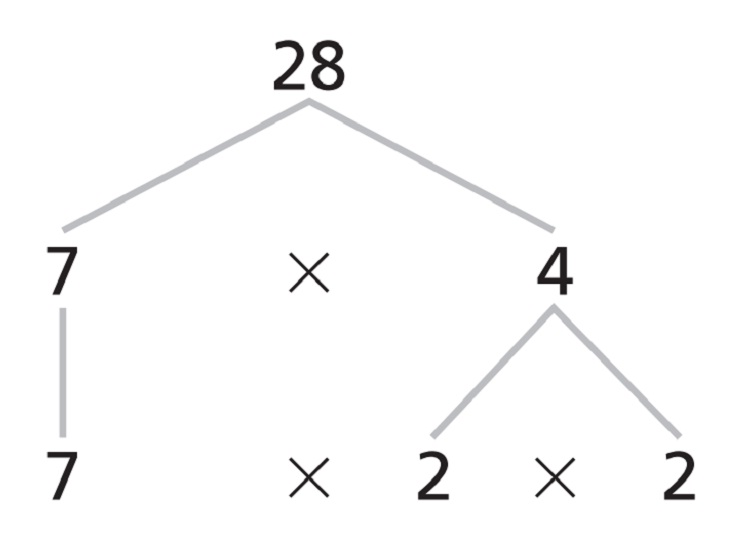﻿ Calculate Prime Factors of a Number | Calculators4U# Prime Factors Calculator

Use our prime factor calculator, a free online conversion tool to calculate and perform prime factorization of a number. A prime number is a whole number greater than one not divisible without a remainder by any whole number other than itself and one. The prime factors of a value are all of the prime numbers that will exactly divide the given value.#### Definition and Facts About Prime Factors

Any composite number can be written as a product using its factors. The Prime Factorization of a number is the product of prime factors equal to that number.

#### Prime Factorization Example

You can use factor trees to find the prime factorization of a number. For example, to make a factor tree for 28, begin by choosing two numberswhose product is 28. Continue dividing each number into two factors untileach of the branches ends in a prime number. The prime factorization of 28 is 2 x 2 x 7, or 22 x 7.